# emd

Empirical mode decomposition

## Syntax

``[imf,residual] = emd(x)``
``[imf,residual,info] = emd(x)``
``[___] = emd(___,Name,Value)``
``emd(___)``

## Description

example

````[imf,residual] = emd(x)` returns intrinsic mode functions `imf` and residual signal `residual` corresponding to the empirical mode decomposition of `x`. Use `emd` to decompose and simplify complicated signals into a finite number of intrinsic mode functions required to perform Hilbert spectral analysis.```

example

````[imf,residual,info] = emd(x)` returns additional information `info` on IMFs and residual signal for diagnostic purposes.```

example

````[___] = emd(___,Name,Value)` performs the empirical mode decomposition with additional options specified by one or more `Name,Value` pair arguments.```

example

````emd(___)` plots the original signal, IMFs, and residual signal as subplots in the same figure.```

## Examples

collapse all

Load and visualize a nonstationary continuous signal composed of sinusoidal waves with a distinct change in frequency. The vibration of a jackhammer and the sound of fireworks are examples of nonstationary continuous signals. The signal is sampled at a rate `fs`.

```load('sinusoidalSignalExampleData.mat','X','fs') t = (0:length(X)-1)/fs; plot(t,X) xlabel('Time(s)')```The mixed signal contains sinusoidal waves with different amplitude and frequency values.

To create the Hilbert spectrum plot, you need the intrinsic mode functions (IMFs) of the signal. Perform empirical mode decomposition to compute the IMFs and residuals of the signal. Since the signal is not smooth, specify '`pchip`' as the interpolation method.

`[imf,residual,info] = emd(X,'Interpolation','pchip');`

The table generated in the command window indicates the number of sift iterations, the relative tolerance, and the sift stop criterion for each generated IMF. This information is also contained in `info`. You can hide the table by adding the `'Display',0` name value pair.

Create the Hilbert spectrum plot using the `imf` components obtained using empirical mode decomposition.

`hht(imf,fs)`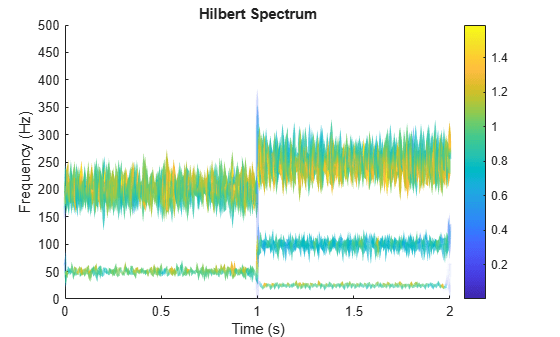The frequency versus time plot is a sparse plot with a vertical color bar indicating the instantaneous energy at each point in the IMF. The plot represents the instantaneous frequency spectrum of each component decomposed from the original mixed signal. Three IMFs appear in the plot with a distinct change in frequency at 1 second.

This trigonometric identity presents two different views of the same physical signal:

$\frac{5}{2}\mathrm{cos2}\pi {\mathit{f}}_{1}\mathit{t}+\frac{1}{4}\left(\mathrm{cos2}\pi \left({\mathit{f}}_{1}+{\mathit{f}}_{2}\right)t+\mathrm{cos2}\pi \left({\mathit{f}}_{1}-{\mathit{f}}_{2}\right)t\right)=\left(2+{\mathrm{cos}}^{2}\pi {\mathit{f}}_{2}\mathit{t}\right)\mathrm{cos2}\pi {\mathit{f}}_{1}\mathit{t}$.

Generate two sinusoids, `s` and `z`, such that `s` is the sum of three sine waves and `z` is a single sine wave with a modulated amplitude. Verify that the two signals are equal by calculating the infinity norm of their difference.

```t = 0:1e-3:10; omega1 = 2*pi*100; omega2 = 2*pi*20; s = 0.25*cos((omega1-omega2)*t) + 2.5*cos(omega1*t) + 0.25*cos((omega1+omega2)*t); z = (2+cos(omega2/2*t).^2).*cos(omega1*t); norm(s-z,Inf) ```
```ans = 3.2729e-13 ```

Plot the sinusoids and select a 1-second interval starting at 2 seconds.

```plot(t,[s' z']) xlim([2 3]) xlabel('Time (s)') ylabel('Signal')```Obtain the spectrogram of the signal. The spectrogram shows three distinct sinusoidal components. Fourier analysis sees the signals as a superposition of sine waves.

`pspectrum(s,1000,'spectrogram','TimeResolution',4)`Use `emd` to compute the intrinsic mode functions (IMFs) of the signal and additional diagnostic information. The function by default outputs a table that indicates the number of sifting iterations, the relative tolerance, and the sifting stop criterion for each IMF. Empirical mode decomposition sees the signal as `z`.

`[imf,~,info] = emd(s);`

The number of zero crossings and local extrema differ by at most one. This satisfies the necessary condition for the signal to be an IMF.

`info.NumZerocrossing - info.NumExtrema`
```ans = 1 ```

Plot the IMF and select a 0.5-second interval starting at 2 seconds. The IMF is an AM signal because `emd` views the signal as amplitude modulated.

```plot(t,imf) xlim([2 2.5]) xlabel('Time (s)') ylabel('IMF')```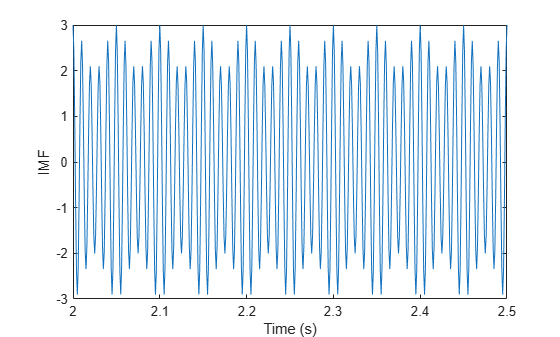Simulate a vibration signal from a damaged bearing. Perform empirical mode decomposition to visualize the IMFs of the signal and look for defects.

A bearing with a pitch diameter of 12 cm has eight rolling elements. Each rolling element has a diameter of 2 cm. The outer race remains stationary as the inner race is driven at 25 cycles per second. An accelerometer samples the bearing vibrations at 10 kHz.

```fs = 10000; f0 = 25; n = 8; d = 0.02; p = 0.12;```The vibration signal from the healthy bearing includes several orders of the driving frequency.

```t = 0:1/fs:10-1/fs; yHealthy = [1 0.5 0.2 0.1 0.05]*sin(2*pi*f0*[1 2 3 4 5]'.*t)/5;```

A resonance is excited in the bearing vibration halfway through the measurement process.

`yHealthy = (1+1./(1+linspace(-10,10,length(yHealthy)).^4)).*yHealthy;`

The resonance introduces a defect in the outer race of the bearing that results in progressive wear. The defect causes a series of impacts that recur at the ball pass frequency outer race (BPFO) of the bearing:

`$BPFO=\frac{1}{2}n{f}_{0}\left[1-\frac{d}{p}\mathrm{cos}\theta \right],$`

where ${f}_{0}$ is the driving rate, $n$ is the number of rolling elements, $d$ is the diameter of the rolling elements, $p$ is the pitch diameter of the bearing, and $\theta$ is the bearing contact angle. Assume a contact angle of 15° and compute the BPFO.

```ca = 15; bpfo = n*f0/2*(1-d/p*cosd(ca));```

Use the `pulstran` function to model the impacts as a periodic train of 5-millisecond sinusoids. Each 3 kHz sinusoid is windowed by a flat top window. Use a power law to introduce progressive wear in the bearing vibration signal.

```fImpact = 3000; tImpact = 0:1/fs:5e-3-1/fs; wImpact = flattopwin(length(tImpact))'/10; xImpact = sin(2*pi*fImpact*tImpact).*wImpact; tx = 0:1/bpfo:t(end); tx = [tx; 1.3.^tx-2]; nWear = 49000; nSamples = 100000; yImpact = pulstran(t,tx',xImpact,fs)/5; yImpact = [zeros(1,nWear) yImpact(1,(nWear+1):nSamples)];```

Generate the BPFO vibration signal by adding the impacts to the healthy signal. Plot the signal and select a 0.3-second interval starting at 5.0 seconds.

```yBPFO = yImpact + yHealthy; xLimLeft = 5.0; xLimRight = 5.3; yMin = -0.6; yMax = 0.6; plot(t,yBPFO) hold on [limLeft,limRight] = meshgrid([xLimLeft xLimRight],[yMin yMax]); plot(limLeft,limRight,'--') hold off```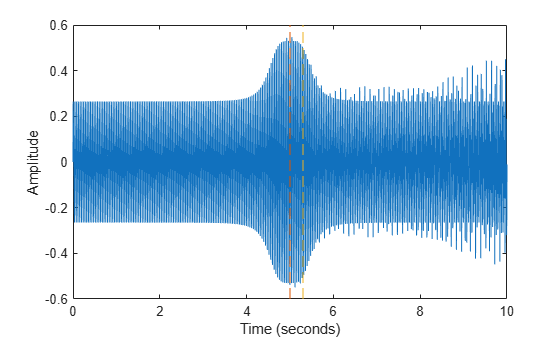Zoom in on the selected interval to visualize the effect of the impacts.

`xlim([xLimLeft xLimRight])`Add white Gaussian noise to the signals. Specify a noise variance of $1/15{0}^{2}$.

```rn = 150; yGood = yHealthy + randn(size(yHealthy))/rn; yBad = yBPFO + randn(size(yHealthy))/rn; plot(t,yGood,t,yBad) xlim([xLimLeft xLimRight]) legend('Healthy','Damaged')```Use `emd` to perform an empirical mode decomposition of the healthy bearing signal. Compute the first five intrinsic mode functions (IMFs). Use the `'Display'` name-value pair to show a table with the number of sifting iterations, the relative tolerance, and the sifting stop criterion for each IMF.

`imfGood = emd(yGood,'MaxNumIMF',5,'Display',1);`
```Current IMF | #Sift Iter | Relative Tol | Stop Criterion Hit 1 | 3 | 0.016207 | SiftMaxRelativeTolerance 2 | 3 | 0.081827 | SiftMaxRelativeTolerance 3 | 6 | 0.14991 | SiftMaxRelativeTolerance 4 | 1 | 0.011853 | SiftMaxRelativeTolerance 5 | 2 | 0.015302 | SiftMaxRelativeTolerance Decomposition stopped because maximum number of intrinsic mode functions was extracted. ```

Use `emd` without output arguments to visualize the first three modes and the residual.

`emd(yGood,'MaxNumIMF',5)`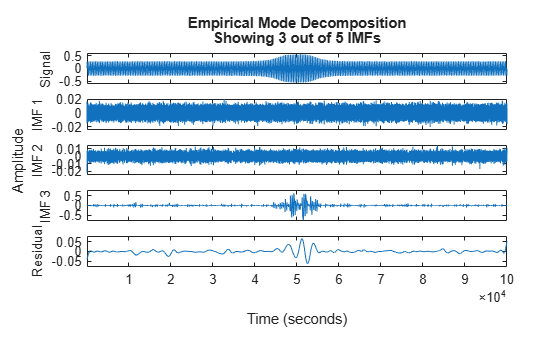Compute and visualize the IMFs of the defective bearing signal. The first empirical mode reveals the high-frequency impacts. This high-frequency mode increases in energy as the wear progresses. The third mode shows the resonance in the vibration signal.

`imfBad = emd(yBad,'MaxNumIMF',5,'Display',1);`
```Current IMF | #Sift Iter | Relative Tol | Stop Criterion Hit 1 | 2 | 0.042507 | SiftMaxRelativeTolerance 2 | 4 | 0.011889 | SiftMaxRelativeTolerance 3 | 4 | 0.19587 | SiftMaxRelativeTolerance 4 | 1 | 0.016427 | SiftMaxRelativeTolerance 5 | 2 | 0.026917 | SiftMaxRelativeTolerance Decomposition stopped because maximum number of intrinsic mode functions was extracted. ```
`emd(yBad,'MaxNumIMF',5)`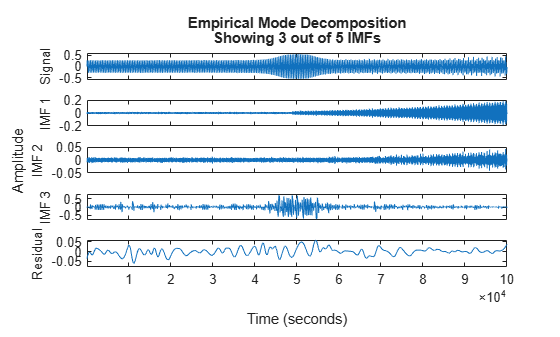The next step in the analysis is to compute the Hilbert spectrum of the extracted IMFs. For more details, see the Compute Hilbert Spectrum of Vibration Signal example.

Load and visualize a nonstationary continuous signal composed of sinusoidal waves with a distinct change in frequency. The vibration of a jackhammer and the sound of fireworks are examples of nonstationary continuous signals. The signal is sampled at a rate `fs`.

```load('sinusoidalSignalExampleData.mat','X','fs') t = (0:length(X)-1)/fs; plot(t,X) xlabel('Time(s)')```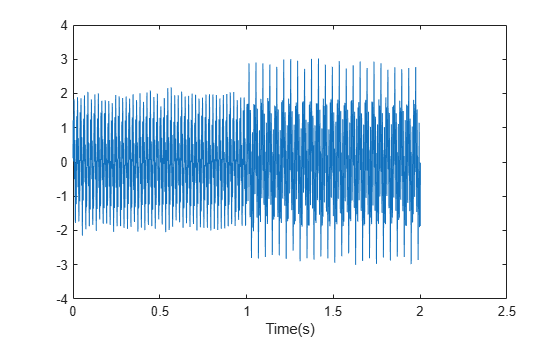The mixed signal contains sinusoidal waves with different amplitude and frequency values.

Perform empirical mode decomposition to plot the intrinsic mode functions and residual of the signal. Since the signal is not smooth, specify '`pchip`' as the interpolation method.

`emd(X,'Interpolation','pchip','Display',1)`
```Current IMF | #Sift Iter | Relative Tol | Stop Criterion Hit 1 | 2 | 0.026352 | SiftMaxRelativeTolerance 2 | 2 | 0.0039573 | SiftMaxRelativeTolerance 3 | 1 | 0.024838 | SiftMaxRelativeTolerance 4 | 2 | 0.05929 | SiftMaxRelativeTolerance 5 | 2 | 0.11317 | SiftMaxRelativeTolerance 6 | 2 | 0.12599 | SiftMaxRelativeTolerance 7 | 2 | 0.13802 | SiftMaxRelativeTolerance 8 | 3 | 0.15937 | SiftMaxRelativeTolerance 9 | 2 | 0.15923 | SiftMaxRelativeTolerance Decomposition stopped because the number of extrema in the residual signal is less than the 'MaxNumExtrema' value. ````emd` generates an interactive plot with the original signal, the first 3 IMFs, and the residual. The table generated in the command window indicates the number of sift iterations, the relative tolerance, and the sift stop criterion for each generated IMF. You can hide the table by removing the `'Display'` name-value pair or specifying it as `0`.

Right-click on the white space in the plot to open the IMF selector window. Use IMF selector to selectively view the generated IMFs, the original signal, and the residual.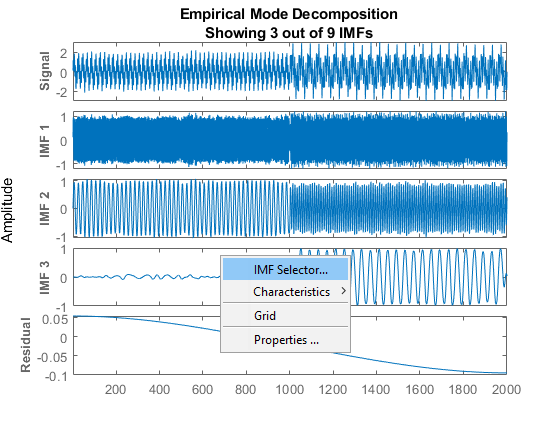Select the IMFs to be displayed from the list. Choose whether to display the original signal and residual on the plot.The selected IMFs are now displayed on the plot.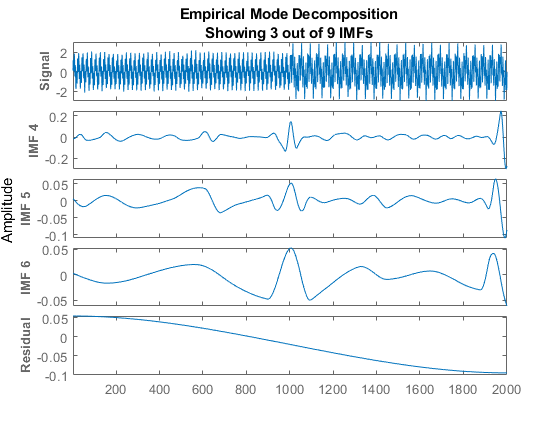Use the plot to visualize individual components decomposed from the original signal along with the residual. Note that the residual is computed for the total number of IMFs, and does not change based on the IMFs selected in the IMF selector window.

## Input Arguments

collapse all

Time-domain signal, specified as a real-valued vector, or a single-variable timetable with a single column. If `x` is a timetable, `x` must contain increasing, finite row times.

### Name-Value Pair Arguments

Specify optional comma-separated pairs of `Name,Value` arguments. `Name` is the argument name and `Value` is the corresponding value. `Name` must appear inside quotes. You can specify several name and value pair arguments in any order as `Name1,Value1,...,NameN,ValueN`.

Example: `'MaxNumIMF',5`

Cauchy-type convergence criterion, specified as the comma-separated pair consisting of `'SiftRelativeTolerance'` and a positive scalar. `SiftRelativeTolerance` is one of the sifting stop criteria, that is, sifting stops when the current relative tolerance is less than `SiftRelativeTolerance`. For more information, see Sift Relative Tolerance.

Maximum number of sifting iterations, specified as the comma-separated pair consisting of `'SiftMaxIterations'` and a positive scalar integer. `SiftMaxIterations` is one of the sifting stop criteria, that is, sifting stops when the current number of iterations is larger than `SiftMaxIterations`.

`SiftMaxIterations` can be specified using only positive whole numbers.

Maximum number of IMFs extracted, specified as the comma-separated pair consisting of `'MaxNumIMF'` and a positive scalar integer. `MaxNumIMF` is one of the decomposition stop criteria, that is, decomposition stops when number of IMFs generated is equal to `MaxNumIMF`.

`MaxNumIMF` can be specified using only positive whole numbers.

Maximum number of extrema in the residual signal, specified as the comma-separated pair consisting of `'MaxNumExtrema'` and a positive scalar integer. `MaxNumExtrema` is one of the decomposition stop criteria, that is, decomposition stops when number of extrema is less than `MaxNumExtrema`.

`MaxNumExtrema` can be specified using only positive whole numbers.

Signal to residual energy ratio, specified as the comma-separated pair consisting of `'MaxEnergyRatio'` and a scalar. `MaxEnergyRatio` is the ratio of the energy of the signal at the beginning of sifting and the average envelope energy. `MaxEnergyRatio` is one of the decomposition stop criteria, that is, decomposition stops when current energy ratio is larger than `MaxEnergyRatio`. For more information, see Energy Ratio.

Interpolation method for envelope construction, specified as the comma-separated pair consisting of `'Interpolation'` and either `'spline'` or `'pchip'`.

Specify `Interpolation` as:

• `'spline'`, if `x` is a smooth signal

• `'pchip'`, if `x` is a nonsmooth signal

`'spline'` interpolation method uses cubic splines, while `'pchip'` uses piecewise-cubic Hermite interpolating polynomials.

Toggle information display in the command window, specified as the comma-separated pair consisting of `'Display'` and either 0 or 1. The table generated in the command window indicates the number of sift iterations, the relative tolerance, and the sift stop criterion for each generated IMF. Specify `Display` as 1 to show the table or 0 to hide the table.

## Output Arguments

collapse all

Intrinsic mode function (IMF), returned as a matrix or timetable. Each IMF is an amplitude and frequency modulated signal with positive and slowly varying envelopes. To perform spectral analysis of a signal, you can apply the Hilbert-Huang transform to its IMFs. See `hht` and Intrinsic Mode Functions.

`imf` is returned as:

• A matrix whose each column is an `imf`, when `x` is a vector

• A timetable, when `x` is a single data column timetable

Residual of the signal, returned as a column vector or a single data column timetable. `residual` represents the portion of the original signal `x` not decomposed by `emd`.

`residual` is returned as:

• A column vector, when `x` is a vector.

• A single data column timetable, when `x` is a single data column timetable.

Additional information for diagnostics, returned as a structure with the following fields:

• `NumIMF` — Number of IMFs extracted

`NumIMF` is a vector from 1 to N, where N is the number of IMFs. If no IMFs are extracted, `NumIMF` is empty.

• `NumExtrema` — Number of extrema in each IMF

`NumExtrema` is a vector equal in length to the number of IMFs. The kth element of `NumExtrema` is the number of extrema found in the kth IMF. If no IMFs are extracted, `NumExtrema` is empty.

• `NumZerocrossing` — Number of zero crossings in each IMF

Number of zero crossings in each IMF. `NumZerocrossing` is a vector equal in length to the number of IMFs. The kth element of `NumZerocrossing` is the number of zero crossings in the kth IMF. If no IMFs are extracted, `NumZerocrossing` is empty.

• `NumSifting` — Number of sifting iterations used to extract each IMF

`NumSifting` is a vector equal in length to the number of IMFs. The kth element of `NumSifting` is the number of sifting iterations used in the extraction of the kth IMF. If no IMFs are extracted, `NumSifting` is empty.

• `MeanEnvelopeEnergy` — Energy of the mean of the upper and lower envelopes obtained for each IMF

If `UE` is the upper envelope and `LE` is the lower envelope, `MeanEnvelopeEnergy` is `mean(((LE+UL)/2).^2)`. `MeanEnvelopeEnergy` is a vector equal in length to the number of IMFs. The kth element of `MeanEnvelopeEnergy` is the mean envelope energy for the kth IMF. If no IMFs are extracted, `MeanEnvelopeEnergy` is empty.

• `RelativeTolerance` — Final relative tolerance of the residual for each IMF

The relative tolerance is defined as the ratio of the squared 2-norm of the difference between the residual from the previous sifting step and the residual from the current sifting step to the squared 2-norm of the residual from the ith sifting step. The sifting process stops when `RelativeTolerance` is less than `SiftRelativeTolerance`. For additional information, see Sift Relative Tolerance. `RelativeTolerance` is a vector equal in length to the number of IMFs. The kth element of `RelativeTolerance` is the final relative tolerance obtained for the kth IMF. If no IMFs are extracted, `RelativeTolerance` is empty.

collapse all

### Empirical Mode Decomposition

The empirical mode decomposition (EMD) algorithm decomposes a signal x(t) into intrinsic mode functions (IMFs) and a residual in an iterative process. The core component of the algorithm involves sifting a function x(t) to obtain a new function Y(t):

• First find the local minima and maxima of x(t).

• Then use the local extrema to construct lower and upper envelopes s(t) and s+(t), respectively, of x(t). Form the mean of the envelopes, m(t).

• Subtract the mean from x(t) to obtain the residual: Y(t) = x(t) − m(t).

An overview of the decomposition is as follows:

1. To begin, let r0(t) = x(t), where x(t) is the initial signal, and let i = 0.

2. Before sifting, check ri(t):

1. Find the total number (TN) of local extrema of ri(t).

2. Find the energy ratio (ER) of ri(t) (see Energy Ratio).

3. If (ER > `MaxEnergyRatio`) or (TN < `MaxNumExtrema`) or (number of IMFs > `MaxNumIMF`) then stop the decomposition.

4. Let ri,Prev(t) = ri(t).

5. Sift ri,Prev(t) to obtain ri,Cur(t).

6. Check ri,Cur(t)

1. Find the relative tolerance (RT) of ri,Cur(t) (see Sift Relative Tolerance).

2. Get current sift iteration number (IN).

7. If (RT < `SiftRelativeTolerance`) or (IN > `SiftMaxIterations`) then stop sifting. An IMF has been found: IMFi(t) = ri,Cur(t). Otherwise, let ri,Prev(t) = ri,Cur(t) and go to Step 5.

8. Let ri+1(t) = ri(t) − ri,Cur(t).

For additional information, see  and .

### Intrinsic Mode Functions

The EMD algorithm decomposes, via an iterative sifting process, a signal x(t) into IMFs imfi(t) and a residual rN(t):

`$X\left(t\right)=\sum _{i=1}^{N}{\text{IMF}}_{i}\left(t\right)+{r}_{N}\left(t\right)$`

When first introduced by Huang et al. , an IMF was defined to be a function with two characteristics:

• The number of local extrema — the total number of local minima and local maxima — and the number of zero crossings differ by at most one.

• The mean value of the upper and lower envelopes constructed from the local extrema is zero.

However, as noted in , sifting until a strict IMF is obtained can result in IMFs that have no physical significance. Specifically, sifting until the number of zero crossings and local extrema differ by at most one can result in pure-tone like IMFs, in other words, functions very similar to what would be obtained by projection on the Fourier basis. This situation is precisely what EMD strives to avoid, preferring AM-FM modulated components for their physical significance.

Reference  proposes options to obtain physically meaningful results. The `emd` function relaxes the original IMF definition by using Sift Relative Tolerance, a Cauchy-type stop criterion. The `emd` function iterates to extract natural AM-FM modes. The IMFs generated may fail to satisfy the local extrema-zero crossings criteria. See Zero Crossings and Extrema in Intrinsic Mode Function of Sinusoid.

### Sift Relative Tolerance

Sift Relative Tolerance is a Cauchy-type stop criterion proposed in . Sifting stops when current relative tolerance is less than `SiftRelativeTolerance`. The current relative tolerance is defined as

Because the Cauchy criterion does not directly count the number of zero crossings and local extrema, it is possible that the IMFs returned by the decomposition do not satisfy the strict definition of an intrinsic mode function. In those cases, you can try reducing the value of the `SiftRelativeTolerance` from its default value. See  for a detailed discussion of stopping criteria. The reference also discusses the advantages and disadvantages of insisting on strictly defined IMFs in empirical mode decomposition.

### Energy Ratio

Energy ratio is the ratio of the energy of the signal at the beginning of sifting and the average envelope energy . Decomposition stops when current energy ratio is larger than `MaxEnergyRatio`. For the ith IMF, the energy ratio is defined as

 Huang, Norden E., Zheng Shen, Steven R. Long, Manli C. Wu, Hsing H. Shih, Quanan Zheng, Nai-Chyuan Yen, Chi Chao Tung, and Henry H. Liu. “The Empirical Mode Decomposition and the Hilbert Spectrum for Nonlinear and Non-Stationary Time Series Analysis.” Proceedings of the Royal Society of London. Series A: Mathematical, Physical and Engineering Sciences 454, no. 1971 (March 8, 1998): 903–95. https://doi.org/10.1098/rspa.1998.0193.

 Rato, R.T., M.D. Ortigueira, and A.G. Batista. “On the HHT, Its Problems, and Some Solutions.” Mechanical Systems and Signal Processing 22, no. 6 (August 2008): 1374–94. https://doi.org/10.1016/j.ymssp.2007.11.028.

 Rilling, Gabriel, Patrick Flandrin, and Paulo Gonçalves. "On Empirical Mode Decomposition and Its Algorithms." IEEE-EURASIP Workshop on Nonlinear Signal and Image Processing 2003. NSIP-03. Grado, Italy. 8–11.

 Wang, Gang, Xian-Yao Chen, Fang-Li Qiao, Zhaohua Wu, and Norden E. Huang. “On Intrinsic Mode Function.” Advances in Adaptive Data Analysis 02, no. 03 (July 2010): 277–93. https://doi.org/10.1142/S1793536910000549.